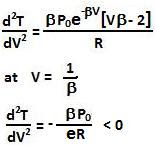## Monday, January 25, 2010

### Irodov Problem 2.11

Since its one mole of an ideal gas we have,a) Also since we know that,Hence we have,Extrema of the temperature will occur when the first derivative is zero.The second derivative is given by,Hence this corresponds to a maximum. So we have,b) Similar to above, using ideal gas equation and given relationship between P and V the we have,Extrema of the temperature will occur when the first derivative is zero.The second derivative is given by,Hence, this corresponds to a maximum. So from (5) and (6) we have,#### 1 comment:

1.this is a bit difficult way to solve q-11.11
can't it be solved by an easier method.